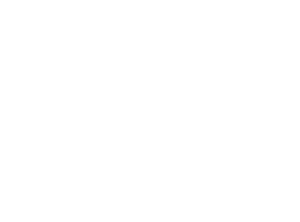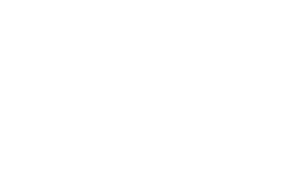# Year 1 Spring 2 Maths Challenge

Hi Year 1 your Spring 2 Maths challenges are as follows:

Red:What is 1 more than 9?

What is 1 more than 7?

What is 1 more than 5?

Yellow and Orange:

What is 1 less than 8?

What is 1 less than 12?

What is is 1 less than 16?

Blue and Green:

What is 1 more than 19?

What is 1 less than 12?

What comes next?A challenge for all of Year 1. Please use the vocabulary below to describe the position of the 3D shapes:

Next to    below     above    inside

1. The sphere is _____________ the pyramid.
2. The cone is ______________ the cylinder.
3. The cube is ______________ the cone.
4. The black dotted lines are ________ the pyramid and cone.

1-below
2-above
3-next to
4-inside

7
11
15

• #### Miss Chahal

Well done Mayar!

3. #### Ouzair Ahmed

For blue and green
What is 1 more than 19 20
What is 1 less
12 11 12 13 14 15 16 17 18 19 20
3D shape change
The sphere is below the pyramid
The cone is above the chider
the cube is next to the cone pyramid and conne
the black dotty line are inside the

• #### Miss Chahal

Well done Ouzir you always work hard to complete your challenges!

Red

9+1= 10
7+1= 8
5+1= 6

Yellow orange

8-1= 7
12-1=11
16-1=15

5. #### Iqra Yuuqow

BLUE group
Green challenge;
1 more than 19= 19+1= 20
1 less than 12= 12-1=11
What comes next after 12+1=13
3D shapes challenge
The sphere is below the pyramid.
The cone is above the cylinder.
The cube is next to the cone.
The black dotted lines are inside the pyramid and cone.

• #### Miss Chahal

Well done Iqra you are correct!

6. #### Abubaker Rauf

1- 1 more than 9 is 10
2- 1 more than 7 is 8
3- 1 more than 5 is 6

The sphere below the pyramid.
The cone is above the cylinder.
The cube is next to the cone.
The black dotted lines are inside the cone and pyramid.

• #### Miss Chahal

Well done Abubaker you have even had a go at the Blue and Green Challenge. Super!

7. #### Yahya Khalifa

Blue group

What is 1 more than 19? 20
What is 1 less than 12? 11
What comes next? What is 1 more than 12? 13

1. The sphere is BELOW the pyramid.
2. The cone is ABOVE the cylinder.
3. The cube is NEXT TO the cone.
4. The black dotted lines are INSIDE the pyramid and cone.

• #### Miss Chahal

Well done Yahya you are correct!

Green
1-20
2-11
3-12
1.below
2.above
3.next to
4.inside

9. #### Rajbir Singh Billing

1 less than 8 = 7
1 less than 12 = 11
1 less than 16 = 15

The sphere is below the pyramid
The cone is above the cylinder
The cube is Next to the cone
The black dotted lines are inside the pyramid and cone

• #### Miss Chahal

Well done Rajbir you have also had a go at the Blue and Green challenge, you are correct!

Green

1. 20
2. 11

what comes next?

1 more than 20 is 21
1 less than 11 is 10

1. Below
2. Above
3. Next to
4. Inside.

11. #### Aamina Bahar

Green challenge;
1 more than 19= 20
1 less than 12= 11
What comes next after 11= 12

3D shapes challenge;
The sphere is below the pyramid.
The cone is above the cylinder.
The cube is next to the cone.
The black dotted lines are inside the pyramid and cone.😀

• #### Miss Chahal

Superstar Aamina! You are correct.

1 less than 8 = 7
1 less than 12 = 11
1 less than 16 = 15
1 more than 19 = 20
1 less than 12 = 11

The sphere is below the pyramid
The cone is above the cylinder
The cube is next to the cone
The black dotted lines are inside the pyramid and cone

• #### Miss Chahal

Well done Adeena you are great at Maths! You are correct!

13. #### Elyas Sultani

yellow what is 1 less than 8? One less than 8=7 what is 1 less than 12? One less than 12 =11 what is 1 less than 16? One less than 16=15

14. #### Bethany Macdonald

1 less than 8=7
1 less than 12 =11
1 less than 16 =15
CHALLENGE
1 The sphere is below the pyramid
2 The cone is above the cylinder
3 The cube is next to the cone
4 The black dotted lines are inside the pyramid and the cone

• #### Miss Chahal

Well done Bethany you are correct!

15. #### Bayleigh Macdonald

1 less than 8 =7
1 less than 12 =11
1 less than 16 =15

The sphere is below the pyramid.
The cone is above the cylinder.
The cube is next to the cone.
The black dotted lines are inside the pyramid and cone.

16. #### Aleena Mirazay

What is 1 more than 19? 19+1=20
What is 1 less than 12? 12-1=11
What comes next? 12

A challenge for all of Year 1. Please use the vocabulary below to describe the position of the 3D shapes:
Next to below above inside
The sphere is ___below___ the pyramid.
The cone is ___above___ the cylinder.
The cube is ___next to___ the cone.
The black dotted lines are __inside___ the pyramid and cone.

17. #### Zaid Mayar

20 is 1 more than 19
11 is 1 less than 12
21 comes after 20 and 12 comes after 11

The sphere is below the pyramid.
The cone is above the cylinder.
The cube is next to the cone.
The black dotted lines are inside the pyramid and cone.

• #### Miss Chahal

Well done Zaid you are a superstar!

18. #### Aleena Husain

What is 1 more than 9 =10
What is 1 more than 7 = 8
What is 1 more than 5 = 6
What is 1 less than 8 = 7
What is 1 less than 12 =11
What is 1 less than 16 =15
What is 1 more than 19 =20
What is 1 more than 22 =23
What is 1 less than 100 =99
What is 1 more than 102 =101
What is 2 less than 23 =20
What is 3 more than 90 =93
What is 3 less than 89 = 92
What is 5 more than 100 = 105
What is 2 less than 26 =24
What is 5 less than 24 =18
What is 1 less than 33 =32

19. #### Merab Muheen Rehman

1more than 19-20-21-22-23-24-25-26-27-28-29-30
1 less than 12-11-10-9-8-7-6-5-4-3-2-1
Challange number 2
1.The sphere is below the pyramid.
2.The cone is above the cylinder.
3.The cube is next to the cone.
4.The black dotted lines are inside the pyramid and cone.

20. #### Siddra Khuram

What is 1 more than 19? 20. What is less than 12? Is 11

21. #### Siddra Khuram

The sphere is the pyramid☺🌼 the cone is 💐 the cylinder the cube🍷is the cone 20+20+20is16

22. #### Christie Weng

What is one more than 19. is 20
what is one less than 12. Is 11

1.The sphere is ______below_______ the pyramid.
2.The cone is _______above_______ the cylinder.
3.The cube is _______next to_______ the cone.
4.The black dotted lines are ____inside____ the pyramid and cone.

• #### Miss Chahal

Well done Christie you are great at completing your challenges!

23. #### Harroop Singh

1 more than 19 is 20
1 less than 12 is 11
And next is 13
The sphere is below the pyramid.
The cone is above the cylinder.
The cube is next to the cone.
The black dotted lines are inside the pyramid and the cone.

24. #### Qasim Khan

What is 1 less than 8?
One less than 8 is 7.
8-1=7
What is 1 less than 12?
One less than 12 is 11.
12-1=11
What is is 1 less than 16?
One less than 16 is 15.
16-1=15

Challenge;
The sphere is below the pyramid.
The cone is above the cylinder.
The cube is next to the cone.
The black dotted lines are inside the pyramid and cone.

• #### Miss Chahal

Well done Qasim you always work hard to complete your challenges!
You have also had a go at the Blue and Green challenge, super!

25. #### Saira Reshma Shafaq

What is 1 more than 19 is 20.
What is 1 Less than 11.
The sphere is below the pyramid.
The cone is above the cylinder.
The cube is next to the cone.
The black dotted lines are inside the pyramid and the cone

26. #### Raihaan Novsarka

1 more then 19 =20,21,22,23,24,25,26,27,28,29,30
One less then 12 =11,10,9,8,7,6,5,4,3,2,1

The sphere is below the pyramid.
The cone is above the cylinder
The cube is next to the cone.
The black dotted lines are inside the pyramid and cone .

1.10
2.8
3.6

28. #### Ravi Jassal

20 is 1 more then 19
11 is 1 less then 12
12 comes after 11,
1:The sphere is below the pyramid
2:The cone is above the cylinder
3:The cube is next to the cone
4:The black dotted lines are inside the pyramid and cone

Green:
What is one more than 19? 19+1=20
What is one less than 12? 12-1=11
What comes next? 12+1=13

1.The sphere is below the pyramid.
2.The cone is above the cylinder.
3.The cube is next to the cone.
4.The black dotted lines are inside the pyramid and cone.

• #### Miss Chahal

For yellow and orange
7
11
15
I try for blue and green
20
11
1.Below
2.Above
3.Next to
4.Inside